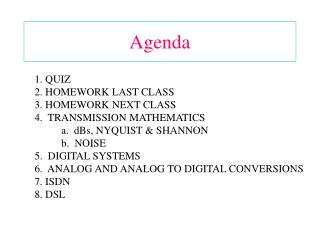DownloadDownload PresentationAgenda

# Agenda

Download Presentation## Agenda

- - - - - - - - - - - - - - - - - - - - - - - - - - - E N D - - - - - - - - - - - - - - - - - - - - - - - - - - -
##### Presentation Transcript

1. Agenda 1. QUIZ 2. HOMEWORK LAST CLASS 3. HOMEWORK NEXT CLASS 4. TRANSMISSION MATHEMATICS a. dBs, NYQUIST & SHANNON b. NOISE 5. DIGITAL SYSTEMS 6. ANALOG AND ANALOG TO DIGITAL CONVERSIONS 7. ISDN 8. DSL

2. Homework Chapter 4: 10, 11, 12, 14, 15, 16, 22, 50, 54, 63 Chapter 5: 1, 3, 6, 7, 9, 14, 17, 32

3. Decibells & Logarithms Converting watts to dB (or milliwatts to dBm): 10 log10 1000 watts = 30 dBw Converting dB to watts (or dBm to milliwatts): 30 dBw = log-1, or log-1 (3) or 10 raised to the 3rd power = 103 = 1000 watts 35 dBw = 103.5 = 3162.3 watts Note: There’s a point between the 3 & 5.

4. Decibells & Logarithms dBW Watts -3 .5 0 1 3 2 6 4 9 8 10 10 20 100 30 1000 40 10000

5. Nyquist 1. Nyquist: The maximum practical data rate (samples) per channel. Max R = 2 H log2 V Logarithmic function to the base 2: For each # V, log V = the exponent to which 2 must be raised to produce V. Then if V = 16, the log2 of V = 4. If V = 2, the log2 of V = 1. Then what is the maximum practical data rate for BPSK signal on a line with a bandwidth of 3000 Hz? What is the maximum practical data rate for a QPSK signal on a line with a bandwidth of 3000 Hz?

6. Shannon Shannon: The maximum theoretical data rate per channel. Max R = CBW x log2 (1 + S/N) [CBW = H in Nyquist Theorem] Then what is the maximum practical data rate for signal with a 30 dB S/N on a line with a bandwidth of 3000 Hz?

7. Noise N = Noise Power = kTB, where B is bandwidth. (Used in Shannon’s Limit) No = Noise Density = kT, where k is Boltzmann’s Constant (-228.6 dBw) (Used in Carrier to Noise ratios, i.e., C/No) T = SNT = System Noise Temperature (Used in radio and satellite link equations, e.g., G/T is a measure of quality in satellite link equations.)

8. Chapter 4 Signals

9. Figure 4-1 Comparison of Analog and Digital Signals

10. Figure 4-2 Example of Periodic Signal

11. Figure 4-3 Example of Aperiodic Signal Aperiodic signals are _____________? Inconsistant

12. Figure 4-4 A Sine Wave

13. Figure 4-5 Amplitude

14. Figure 4-6 Period and Frequency

15. Figure 4-7 Relationship between Different Phases

16. Figure 4-8 Amplitude Change Amplitude relates to ___________? S in S/N

17. Figure 4-10 Phase Change Phase shift relates to ______________? Phase shift keying

18. Figure 4-11 Time and Frequency Domains

19. Time and Frequency Domains for Different Signals Figure 4-12

20. Figure 4-13 A Signal with a DC Component

21. Figure 4-14 Composite Waveform

22. Figure 4-15 Bandwidth

23. Figure 4-16 Example 4.8

24. Figure 4-17 Example 4.9

25. Figure 4-19 Bit Rate and Bit Interval

26. Figure 4-20 Harmonics of a Digital Signal

27. Figure 4-21 Exact and Significant Spectrum

28. Chapter 5 Encoding

29. Figure 5-3 Types of Digital to Digital Encoding

30. Figure 5-5 Types of Polar Encoding

31. Figure 5-6 NRZ-L and NRZ-I Encoding

32. Figure 5-7 RZ Encoding

33. Figure 5-8 Manchester and Diff. Manchester Encoding

34. Figure 5-9 Types of Bipolar Encoding

35. Figure 5-10 Bipolar AMI Encoding

36. Figure 5-15 Analog to Digital Conversion

37. Figure 5-16 PAM

38. Figure 5-17 Quantized PAM Signal

39. Figure 5-19 PCM

40. Figure 5-20 From Analog Signal to PCM Digital Code

41. Figure 5-21 Nyquist Theorem This assumes what?

42. Figure 5-27 FSK

43. Figure 5-28 Baud Rate and Bandwidth in FSK

44. Figure 5-29 PSK Why do you think PSK is better than FSK? Needs less power per bit

45. Figure 5-30 PSK Constellation

46. Figure 5-31 4-PSK

47. Figure 5-32 4-PSK Characteristics

48. Figure 5-33 8-PSK Characteristics

49. Figure 5-34 Baud Rate and Bandwidth in PSK

50. Figure 5-35 4-QAM and 8-QAM Constellations